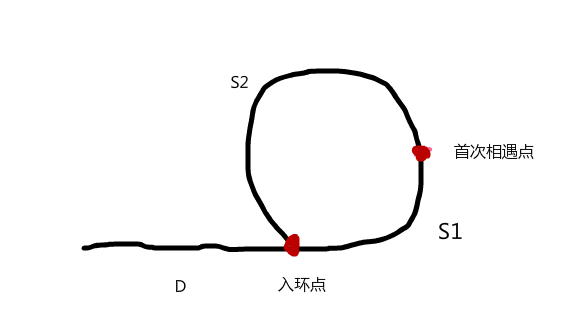# Python 判断链表中是否有环，且找出环的入口节点

hresh 656## 概述## 应用import string

#节点对象
class Node():
def __init__(self, val=None):
self.val = val  #节点数据值
self.next = None    #相连的下一个节点对象

def __str__(self):
return str(self.val)

#单链表
def __init__(self, node=None):

'''
单链表添加元素
:param var:传过来的数据
:return:
'''
node = Node(var)  # 将数据封装为节点
else:
while cur.next != None:
cur = cur.next  #移动游标
cur.next = node

def traversal(self):
'''
遍历输出单链表
:return:
'''
return
else:
while cur != None:
print(cur,end=' ')
cur = cur.next
print()

def fprint_cycle(self, length):
'''
输出有环链表，因为有环，所以如果按照之前的输出方法，会一直输出，所以我们只输出到交叉点
:param length: 无环链表的长度加 1
:return:
'''
for i in range(length):
print(cur, end=' ')
cur = cur.next
print()

def size(self):
'''
链表长度
:return:
'''
count = 0
while cur != None:
count += 1
cur = cur.next
return count

def setCycleList(self, index):
'''
输入索引值，确定哪一点为交叉点，即环入口点
:param index:
:return:
'''
size = self.size()
if size == 0:
print('Empty,can\'t delete')
return

if index <= 0 or index > size-1:
print('Index is error')
return

while cur.next != None:
cur = cur.next

tail_node = cur #获取尾节点

pre = cur
for i in range(index + 1):  #获取链表环的交叉点
pre = cur
cur = cur.next

tail_node.next = pre    #尾节点指向交叉点，组成一个环

def hasCycle(self):
'''
判断单链表是否有环
:return:有环返回True，否则为 False
'''

while p != None and p.next != None:#当p快指针和q慢指针相遇，则说明存在环
p = p.next.next
q = q.next
if p == q:  #此时p指针和q指针同时指向同一个节点
return True
return False

if __name__ == '__main__':

for i,v in enumerate(string.ascii_lowercase):
if i == 12:
break

print("对链表进行遍历")

#构建有环链表

#判断链表是否有环
print('该链表有环！')


    def getCycle_length(self):
'''
计算链表中环的长度
:return:
'''

p_distance = 0
q_distance = 0

R = 0
while p != None and p.next != None:  # 当p快指针和q慢指针相遇，则说明存在环
p = p.next.next
p_distance += 2
q = q.next
q_distance += 1

if p == q:
R = p_distance - q_distance
break
return Rp 指针和 q 指针首次相遇时，各自走的距离为：L(q) = D+S1，L(p) = D+S1+S2+S1

    def get_intersection(self):

s = None
while p != None and p.next != None:  # 当p快指针和q慢指针相遇，则说明存在环
p = p.next.next
q = q.next
if p == q:  # 此时p指针和q指针同时指向同一个节点
s = p
break

while r != s:
r = r.next
s = s.next

e = r
return e